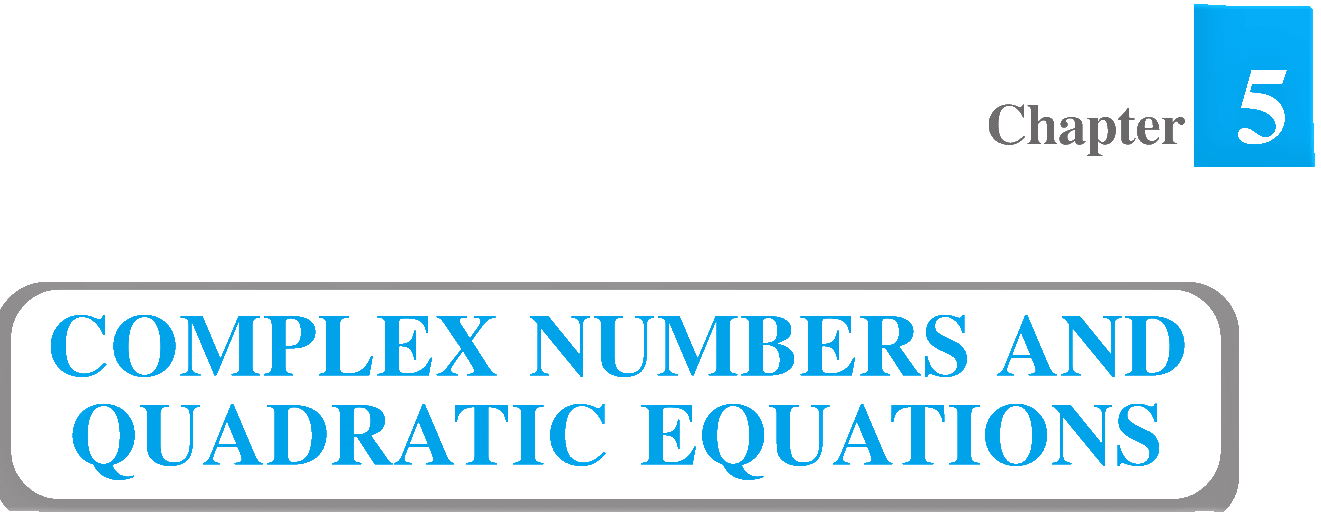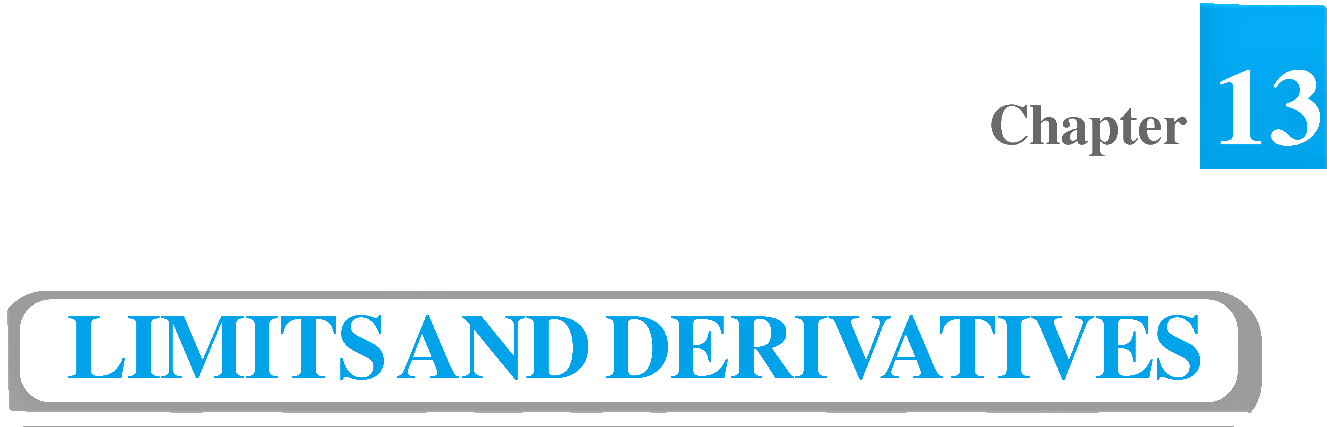# NCERT Solutions for Class 11 Maths

Ashish Kumar – Let’s Learn is Online Educational Platform that provides NCERT Solutions for Class 11 Maths with online expert created animated video tutorials, pdf notes and assignments.

Online Video Lectures having explanations from basic to advance concepts, each every NCERT exercise questions, important questions, supplementary exercise questions and important examples explained by expert teacher. Our deep learning resources covers all the basic concepts, including the advanced concepts from the boards point of view.

## Select ChapterSets and their representations. Empty set. Finite and Infinite sets. Equal sets. Subsets. Subsets of a set of real numbers especially intervals (with notations). Power set. Universal set. Venn diagrams. Union and Intersection of sets. Difference of sets. Complement of a set. Properties of Complement.Ordered pairs. Cartesian product of sets. Number of elements in the Cartesian product of two finite sets. Cartesian product of the set of reals with itself (upto R x R x R). Definition of relation, pictorial diagrams, domain, co-domain and range of a relation. Function as a special type of relation. Pictorial representation of a function, domain, co-domain and range of a function. Real valued functions, domain and range of these functions, constant, identity, polynomial, rational, modulus, signum, exponential, logarithmic and greatest integer functions, with their graphs. Sum, difference, product and quotients of functions.Positive and negative angles. Measuring angles in radians and in degrees and conversion from one measure to another. Definition of trigonometric functions with the help of unit circle. Truth of the identity \sin^{2}x+\cos^{2}x=1 , for all x. Signs of trigonometric functions. Domain and range of trigonometric functions and their graphs. Expressing sin (x ± y) and cos (x ± y) in terms of sinx, siny, cosx & cosy and their simple applications. Deducing identities. Identities related to sin2x, cos2x, tan2x, sin3x, cos3x and tan3x. General solution of trigonometric equations of the type \sin y = \sin a , \cos y = \cos a and \tan y = \tan a .Process of the proof by induction, motivating the application of the method by looking at natural numbers as the least inductive subset of real numbers. The principle of mathematical induction and simple applications.Need for complex numbers, especially √-1 , to be motivated by inability to solve some of the quardratic equations. Algebraic properties of complex numbers. Argand plane and polar representation of complex numbers. Statement of Fundamental Theorem of Algebra, solution of quadratic equations (with real coefficients) in the complex number system. Square root of a complex number.Linear inequalities. Algebraic solutions of linear inequalities in one variable and their representation on the number line. Graphical solution of linear inequalities in two variables. Graphical method of finding a solution of system of linear inequalities in two variables.Fundamental principle of counting. Factorial n. (n!) Permutations and combinations, derivation of formulae for C(n, r) and P(n, r) and their connections, simple applications.History, statement and proof of the binomial theorem for positive integral indices. Pascal’s triangle, General and middle term in binomial expansion, simple applications.Sequence and Series. Arithmetic Progression (A. P.). Arithmetic Mean (A.M.) Geometric Progression (G.P.), general term of a G.P., sum of n terms of a G.P., infinite G.P. and its sum, geometric mean (G.M.), relation between A.M. and G.M. Formulae for the special sums.Brief recall of 2-D from earlier classes, shifting of origin. Slope of a line and angle between two lines. Various forms of equations of a line: parallel to axes, point-slope form, slope-intercept form, two-point form, intercepts form and normal form. General equation of a line. Equation of family of lines passing through the point of intersection of two lines. Distance of a point from a line.Sections of a cone: Circles, ellipse, parabola, hyperbola, a point, a straight line and pair of intersecting lines as a degenerated case of a conic section. Standard equations and simple properties of parabola, ellipse and hyperbola. Standard equation of a circle.Derivative introduced as rate of change both as that of distance function and geometrically, intuitive idea of limit. Definition of derivative, relate it to slope of tangent of the curve, derivative of sum, difference, product and quotient of functions. Derivatives of polynomial and trigonometric functions.Random experiments, outcomes; Sample spaces (set
representation); Event; Occurrence of events, “not’, “and” and “or” events. exhaustive events, mutually exclusive events, Axiomatic (set theoretic) probability connections with the
theories of earlier classes. Probability of an event, probability of “not”, “and” and “or” events.